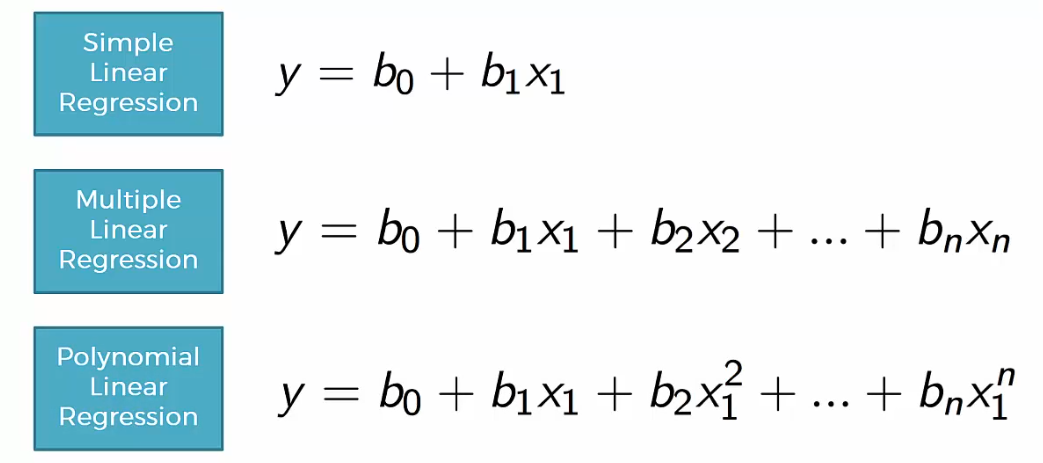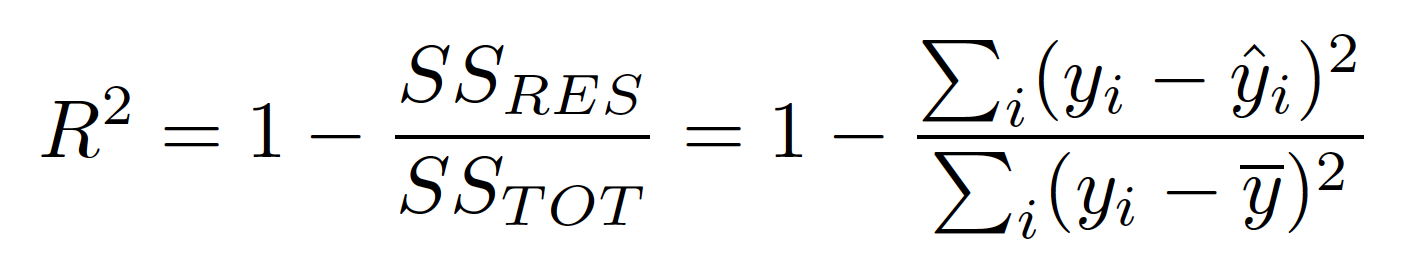## Machine Learning – Part 1/100

1. What is linear regression? What do the terms r-squared and p-value mean? What is the significance of each of these components?

Linear regression is a linear model, e.g. a model that assumes a linear relationship between the input variables (x) and the single output variable (y)There are many variants in Regression and here are some

Simple Regression contains one input variable and one output variable

Multiple Regression contains multiple input variables and one output variable

Polynomial Regression contains polynomial variants of the input variables and one output variable

R-Square value tells how much variation is explained by your model. So, 0.1 R-square means that your model explains 10% of variation within the data. The greater R-square the better the model.P-value tells about the F statistic hypothesis testing of the “fit of the intercept-only model and your model are equal”. So if the p-value is less than the significance level (usually 0.05) then your model fits the data well.

Thus you have four scenarios:

1. Low R-square and Low p-value (p-value <= 0.05)

2. Low R-square and High p-value (p-value > 0.05)

3. High R-square and Low p-value

4. High R-square and High p-value

Interpretation:

1. Means that your model doesn’t explain much of variation of the data but it is significant (better than not having a model)

2. Means that your model doesn’t explain much of variation of the data and it is not significant (worst scenario)

3. Means your model explains a lot of variation within the data and is significant (best scenario)

4. Means that your model explains a lot of variation within the data but is not significant

2. What are the assumptions required for linear regression?

There are four major assumptions:

1. There is a linear relationship between the dependent variables and the regressors, meaning the model you are creating actually fits the data,

2. The errors or residuals of the data are normally distributed and independent from each other

3. There is minimal multi-collinearity between explanatory variables

4. Homoscedasticity – This means the variance around the regression line is the same for all values of the predictor variable

3. What are the drawbacks of linear regression?

1. Assumption of Linearity between independent and dependent variables – In real world, the variables might not show a linear relationship

2. Linear regression is sensitive to outliers

3. Multi-Collinearity – presence of collinearity between the variables will reduce the predictive power of the variables

4. Overfittting – It is easy to overfit your model such that your regression begins to model the random error (noise) in the data, rather than just the relationship between the variables. This most commonly arises when you have too many parameters compared to the number of samples

4. How does multi-collinearity affect the linear regression?

Multicollinearity occurs when some of the independent variables are highly correlated (positively or negatively) with each other.  The presence of multicollinearity does not affect the predictive capability of the model but it does pose a problem in understanding the individual effects of collinear variables on the response variable. It reduces the accuracy of the estimates of the regression coefficients during model building.

5. How do you identify the significance of an independent variable in linear regression?

6. Explain how outliers impact linear regression?

7. What is the role of High leverage points in linear regression?

8. Explain how do you identify the non-linearity in the data ? Also, explain what is the affect of non-linear data on linear regression?

9. What are Residual plots?

10. What is Heteroscedasticity?

11. What is Homoscedasticity?

12.  How do you test correlation between error terms in linear regression?

13. How do you check if your model is significant or not?

14. What are requirements for building a linear regression?

15. Explain Ridge Regression?

16. Explain Lasso Regression?

17. What are L1 and L2 regression? How are they different?

18. Explain cost function in Linear Regression?

19. How do you measure the performance of your model?

20. How is correlation linked to R-Square?

21. Explain R-Square?

23. What is MAE?

24. What is MSE?

25. What is RMSE?

26. Explain the steps in Linear Regression?

27. How do you check if there is a relationship between response and predictor variables?

28. Explain the difference between Correlation and Collinearity?

29. How do you assess the accuracy of  linear regression?

30. How does number of observations influence overfitting?

31. Explain the difference between Regression and Correlation?

32. How should Training accuracy and Testing accuracy be?

33. Explain the role of bias in a model?

34. Explain the role of variance in a model?

35. How do you check if your model is overfitting or underfitting?

36. Explain what steps you would take if you notice your model is overfitting?

37. Explain what steps you would take if you notice your model is underfitting?

38. Explain steps involved in feature selection?

39. Explain the importance of F-test in linear regression?

40. What is curse of dimensionality?

41. How do you remove multi-collinearity in data?

42. What is VIF? How do you calculate it?

43. What are the advantages of  Least Square Estimate?

OLS coefficient or beta estimates are the “Best Linear Unbiased Estimates (BLUE)”, provided the error terms are uncorrelated (no autoregression) and have equal variance (homoscedasticity).